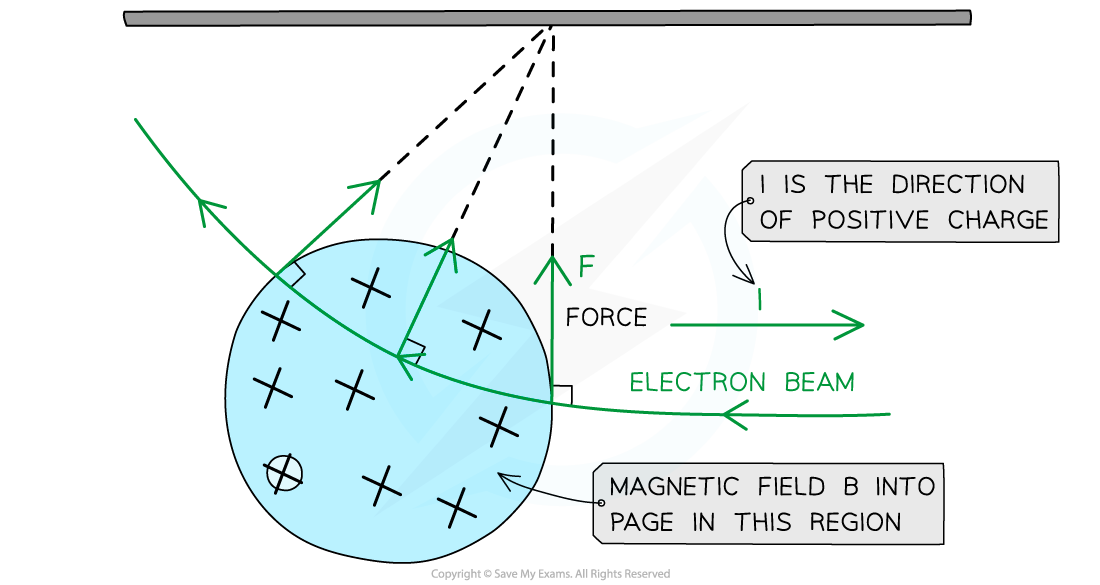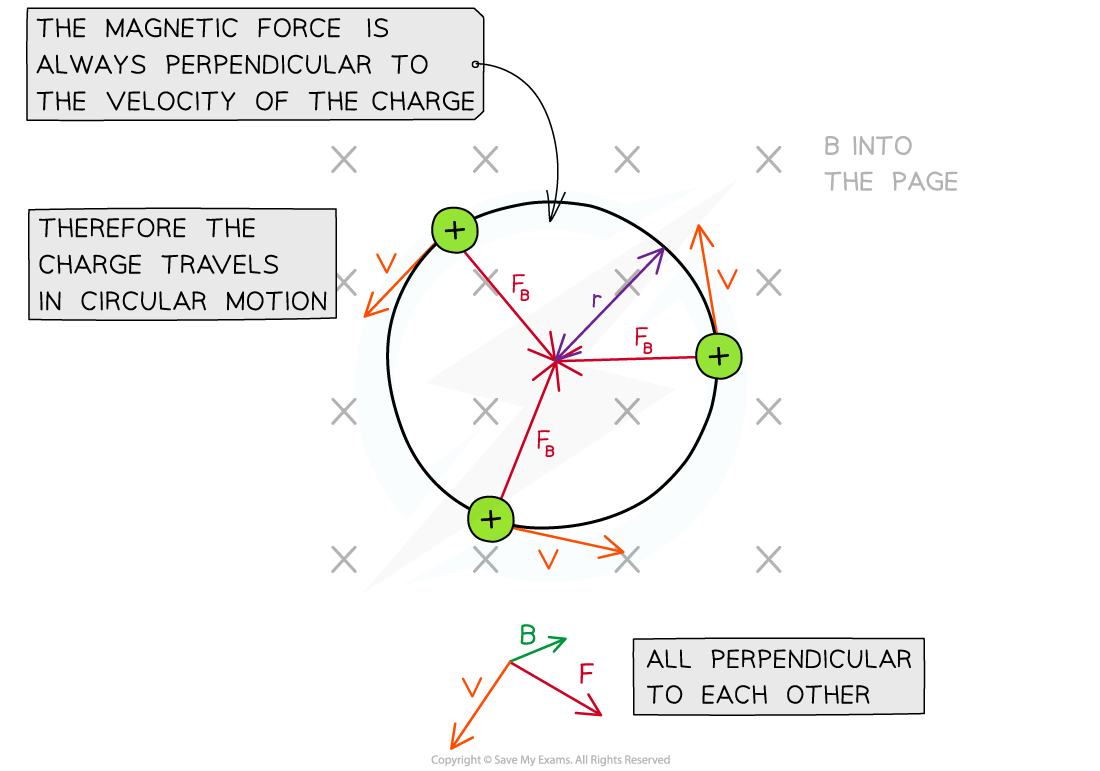# IB DP Physics: HL复习笔记5.4.2 Magnetic Force on a Charge

### Magnetic Force on a Charge

#### Calculating Magnetic Force on a Moving Charge

• The magnetic force on an isolating moving charge, such an electron, is given by the equation:

F = BQv sinθ

• Where:
• F = force on the charge (N)
• B = magnetic flux density (T)
• Q = charge of the particle (C)
• v = speed of the charge (m s-1)
• θ = angle between charge’s velocity and magnetic field (degrees)The force on an isolated moving charge is perpendicular to its motion and the magnetic field B

• Equivalent to the force on a wire, if the magnetic field B is perpendicular to the direction of the charge’s velocity, the equation simplifies to:

F = BQv

• According to Fleming’s left hand rule:
• When an electron enters a magnetic field from the left, and if the magnetic field is directed into the page, then the force on it will be directed upwards
• The equation shows:
• If the direction of the electron changes, the magnitude of the force will change too
• The force due to the magnetic field is always perpendicular to the velocity of the electron
• Note: this is equivalent to circular motion
• Fleming’s left-hand rule can be used again to find the direction of the force, magnetic field and velocity
• The key difference is that the second finger representing current I (direction of positive charge) is now the direction of velocity v of the positive chargeThe electron experiences a force upwards when it travels through the magnetic field between the two poles

#### Worked Example

An electron is moving at 5.3 × 107 m s-1 in a uniform magnetic field of flux density 0.2 T.Calculate the force on the electron when it is moving at 30° to the field, and state the factor it increases by compared to when it travels perpendicular to the field.

Step 1: Write out the known quantities

Speed of the electron, v = 5.3 × 107 m s-1

Charge of an electron, Q = 1.60 × 10-19 C

Magnetic flux density, B = 0.2 T

Angle between electron and magnetic field, θ = 30°

Step 2: Write down the equation for the magnetic force on an isolated particle

F = BQv sinθ

Step 3: Substitute in values, and calculate the force on the electron at 30°

F = (0.2) × (1.60 × 10-19) × (5.3 × 107) × sin(30) = 8.5 × 10-13 N

Step 4: Calculate the electron force when travelling perpendicular to the field

F = BQv = (0.2) × (1.60 × 10-19) × (5.3 × 107) = 1.696 × 10-12 N

Step 5: Calculate the ratio of the perpendicular force to the force at 30°Therefore, the force on the electron is twice as strong when it is moving perpendicular to the field than when it is moving at 30° to the field

#### Motion of a Charged Particle in a Uniform Magnetic Field

• A charged particle in uniform magnetic field which is perpendicular to its direction of motion travels in a circular path
• This is because the magnetic force FB will always be perpendicular to its velocity v
• FB will always be directed towards the centre of the pathA charged particle moves travels in a circular path in a magnetic field

• The magnetic force FB provides the centripetal force on the particle
• Recall the equation for centripetal force:• Where:
• m = mass of the particle (kg)
• v = linear velocity of the particle (m s-1)
• r = radius of the orbit (m)
• Equating this to the force on a moving charged particle gives the equation:• Rearranging for the radius r obtains the equation for the radius of the orbit of a charged particle in a perpendicular magnetic field:• This equation shows that:
• Faster moving particles with speed v move in larger circles (larger r): r  v
• Particles with greater mass m move in larger circles: ∝ m
• Particles with greater charge q move in smaller circles: r ∝ 1 / q
• Particles moving in a strong magnetic field B move in smaller circles: ∝ 1 / B

#### Worked Example

An electron with charge-to-mass ratio of 1.8 × 1011 C kg-1 is travelling at right angles to a uniform magnetic field of flux density 6.2 mT. The speed of the electron is 3.0 × 106 m s-1.Calculate the radius of the circle path of the electron.

Step 1: Write down the known quantitiesMagnetic flux density, B = 6.2 mT

Electron speed, v = 3.0 × 106 m s-

Step 2: Write down the equation for the radius of a charged particle in a perpendicular magnetic fieldStep 3: Substitute in values#### Exam Tip

• Remember not to mix this up with F = BIL!
• F = BIL is for a current carrying conductor
• F = Bqv is for an isolated moving charge (which may be inside a conductor)
• It is important to note that when the moving charge is traveling along the field direction - precisely with or against the field lines - then there is no magnetic force on that charge!